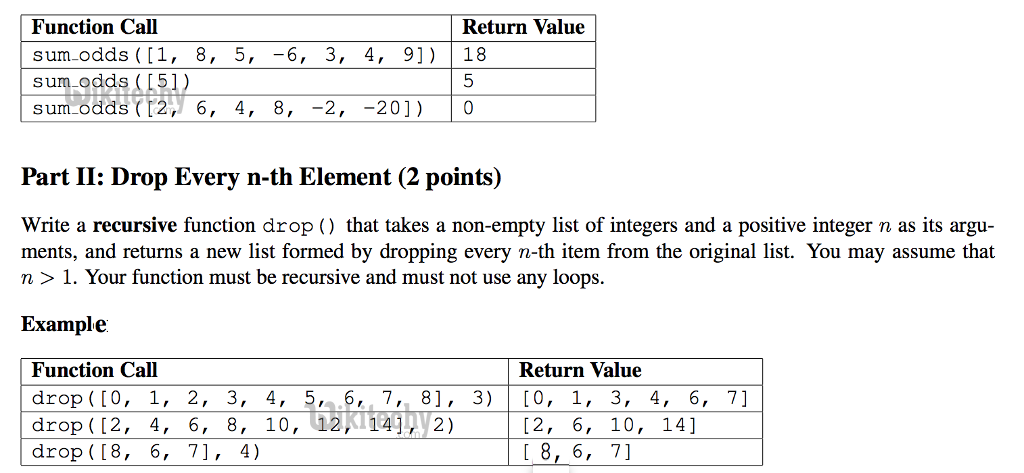# python tutorial - Python question and answer - learn python - python programming

## python interview questions :201

Similar to its sibling the list comprehension, a dictionary comprehension is nothing more than a shorthand way of creating a new dictionary collection.
• Or for Python 3:

## How about summing only odd elements?Learn python - python tutorial - odd - python examples - python programs

## How to truncate division operation?

• The truncating division operator (also known as floor division) truncates the result to an integer and works with both integers and floating-point numbers.
• As of this writing, the true division operator (/) also truncates the result to an integer if the operands are integers. Therefore, 7/4 is 1, not 1.75.
• However, this behavior is scheduled to change in a future version of Python, so you will need to be careful.
• The modulo operator returns the remainder of the division x // y. For example, 7 % 4 is 3.
• For floating-point numbers, the modulo operator returns the floating-point remainder of x // y, which is x  (x // y) * y. For complex numbers, the modulo (%) and truncating division operators (//) are invalid.
• Division of two number like this:

Output:

Output:

## How to Print a list of file in a directory?

• If you want to print a list of files in a directory including the sub-directories. You may want to do it recursively.

Output:

Output:

## How to reverse a string using recursive function?

• In the following recursive code, at every call, the first string will go to the end and stored in stack. So, when there is only one character left as an input string, the code starts rewind and retrieve the character one by one.
• The net effect is it gets the character in reverse. It becomes obvious when we see the printed output below:

Output:

## How to Merge the overlapped range?

• You want to merge all overlapping ranges and return a list of distinct ranges. For example:
• Here is the code:

Output:

## How to Initialize dictionary with list - I ?

• Sometimes If you want to construct dictionary whose values are lists.
• In the following example, we make a dictionary like {'Country': [cities,...], }:

Output:

## How to Initialize dictionary with list - II?

• A little bit simpler problem. If youhave a list of numbers:

Output: